Conge 精进

2019-01-23

# 01 - Introduction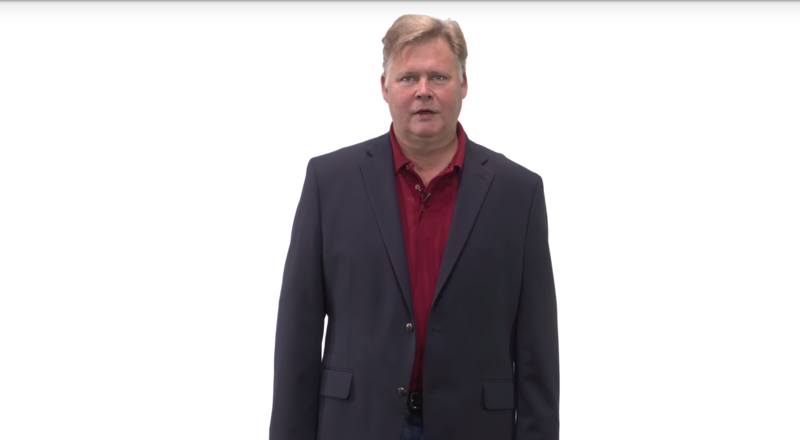supervised regression learning or numerical model:

• using data to build a model that predicts a numerical output based on a set of numerical inputs.

Time: 00:00:23

# 02 - Parametric regression

Parametric regression is a way of building a model where we represent the model with the number of parameters. Example: build a model that will predict how much it will rain today based on changes in barometric pressure.

• if barometric pressure declines -> bad weather or rain.
• And when barometric pressure increases -> good weather coming.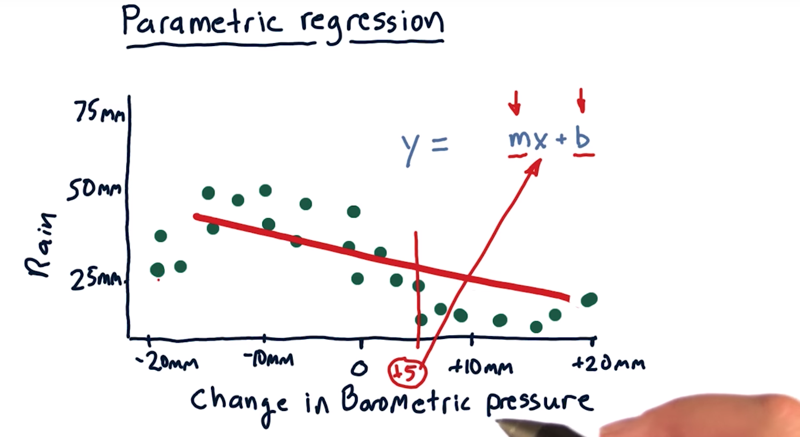• each one of these dots represents one day’s worth of data and we have multiple days worth of data here.
• there’s a general trend of as barometric pressure decreases, we typically have more rain and as it increases we have less rain.
• The classic solution to this problem is to fit a line to the data. (linear regression).
• The equation: y = m*x + b. which is a model fully described by two parameters m and b.
• This model is decent, but it doesn’t track the actual behavior of the data, for instance,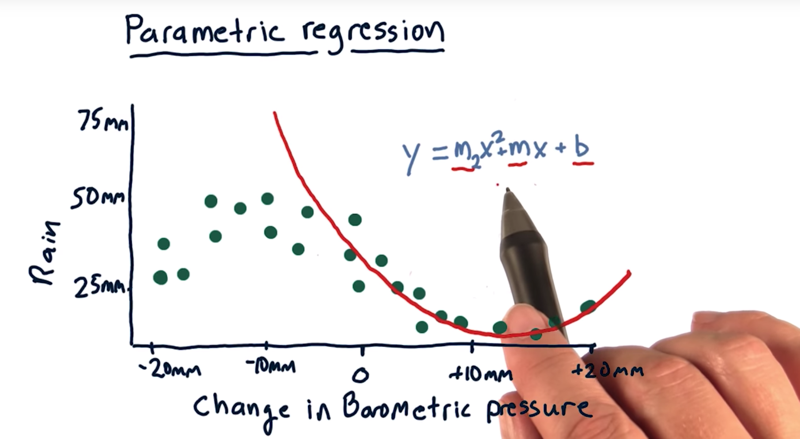• we can fit a polynomial, by adding\ more term x2 and an additional parameter m2.
• we can add more terms.

All of these models are parametric models. In the end, after we learn these models and get our parameters (e.g. m2, m, and b), we can through away the data.

Time: 00:04:12

# 03 - K nearest neighbor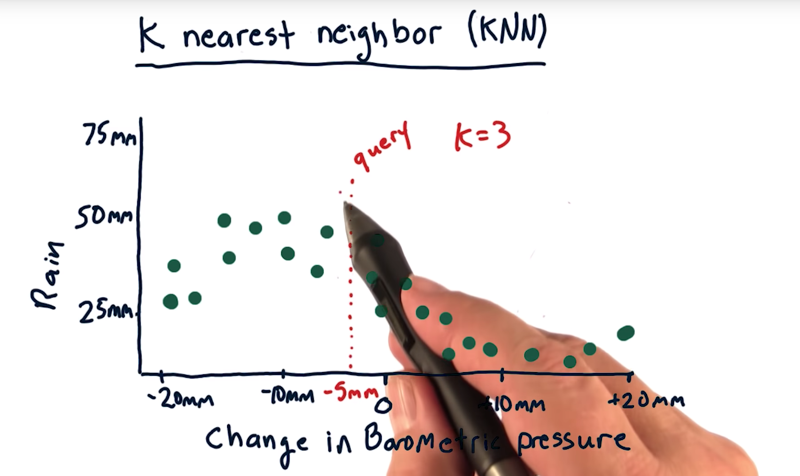Data-centric or instance based approach: keep and use the data when we make a query.

• E.G. 3-NN and query here is at -5 millimeters.
• we will find the 3 nearest historical data points to this query and use them to estimate how much it’s going to rain today

Time: 00:00:45

# 04 - Quiz: How to predict

what should we do with these data points to find that prediction?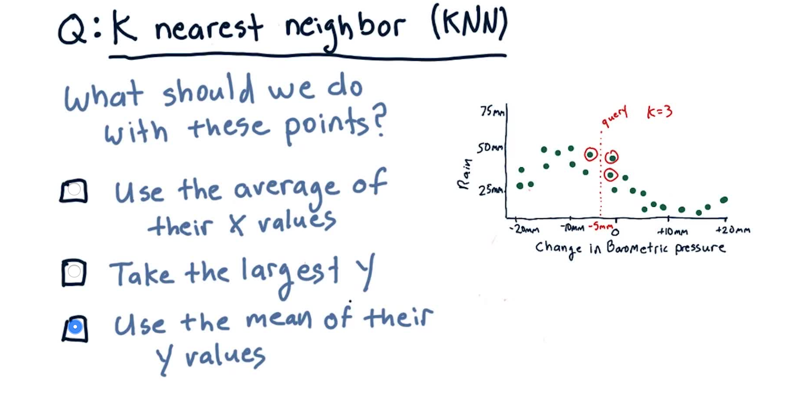• We don’t want to take the largest y, because we want to take advantage of all these votes that we have to get a closer, more correct answer.

Time: 00:00:30

# 05 - Kernel regression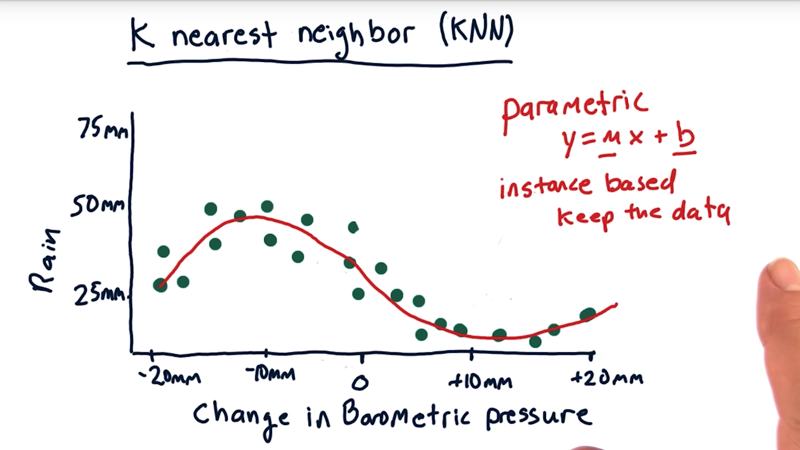• KNN will model the data like the red line, which fits the data where it’s curving.
• it interpolates nicely and smoothly between all the data points.
• The most famous of these methods is K nearest neighbor.
• kernel regression is another.
• The main way that KNN differs from kernel regression is that kernel regression weights the contributions of each of the nearest data points according to how distant they are.
• Where as with KNN, each data point that we consider gets essentially an equal weight.

Time: 00:01:32

# 06 - Parametric vs non-parametric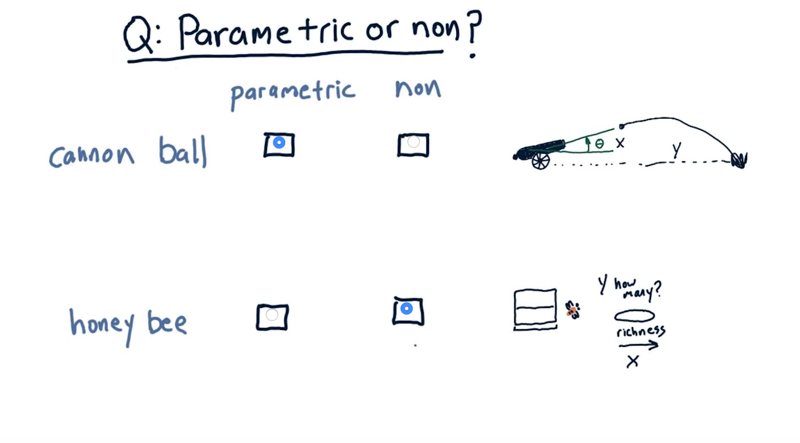• first problem: predicting cannonball distance with cannon aiming angle,
• Second problem: predicting the number of bees a food source will attact given the richness of the food source.

the cannon ball distance can be best estimated using a parametric model, as it follows a well-defined trajectory. On the other hand, the behavior of honey bees can be hard to model mathematically. Therefore, a non-parametric approach would be more suitable.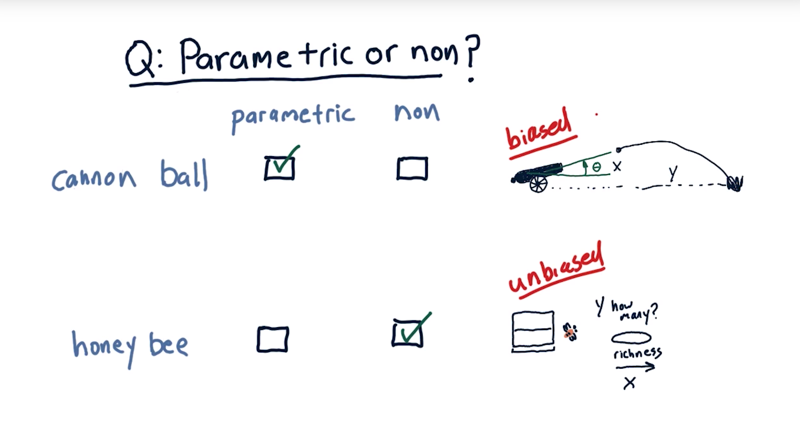• the cannonball model is biased, in the sense that we have an initial guess of what the form of the equation is.
• the bee model is unbiased because we don’t know.

__ the pros and cons of each approach__.

1. parametric approach:
• we don’t have to store the original data, so it’s very space efficient,
• but we can’t easily update the model as more data is gathered.
• training is slow
2. non-parametric approaches, or instance-based: have to store all the data points.
• hard to apply when we have a huge data set,
• but new evidence can be added easily s
• training is fast, but querying is potentially slow.
• nonparametric approaches avoid having to assume a certain type of model so they’re suitable to fit complex patterns

Time: 00:02:47

#07 - Training and testing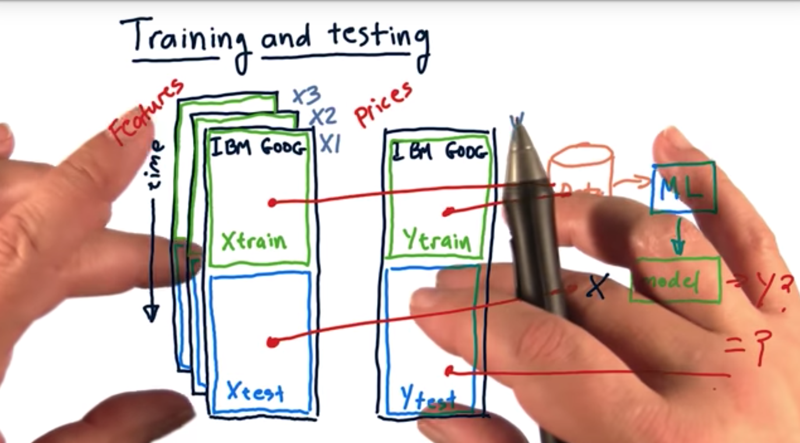• X data: X1, X2, X3, and so on.
• Y data, which we’re trying to predict.
• In order to evaluate our learning algorithms in a scientific manner we need to split this data into at least two sections. A training section and a testing section.

out of sample testing: The procedure of separating testing and training data from one another.

• take our Xtrain data and our Ytrain data, run that through our machine learning algorithm to generate a model.
• Then test the accuracy of that model using Xtest and Ytest data.
• the input to the model is Xtest, and the model gives back Y-predict.
• compare Y-predict data with Ytest data,the more closely the model outputs a Y that reflects this Xtest data, the more accurate the model is.

in this class, our data is time oriented, and we typically split the data up according to time.

• train model on older data and test it on newer data.
• doing the reverse will have certain look-ahead biases.

Time: 00:02:50

# 08 - Learning APIs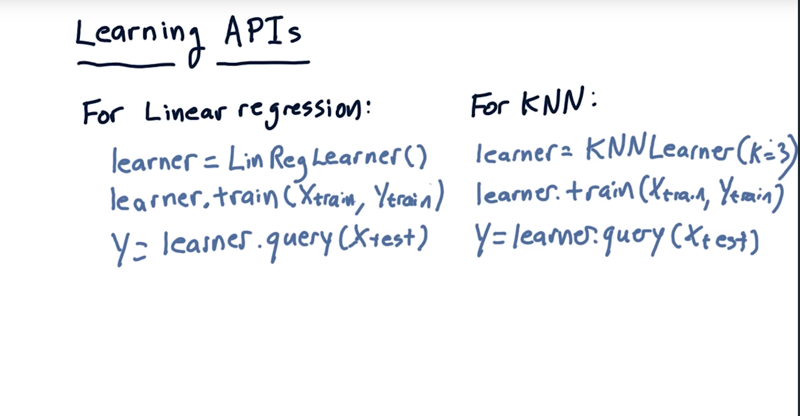When write some machine learning algorithms in the class, let’s standardize on what the application programmer interface ought to look like for the code.

• the learner should have a train method and a query method.

linearregression Learner. KNNLearner: additional argument, K

Time: 00:01:14

# 09 - Example for linear regression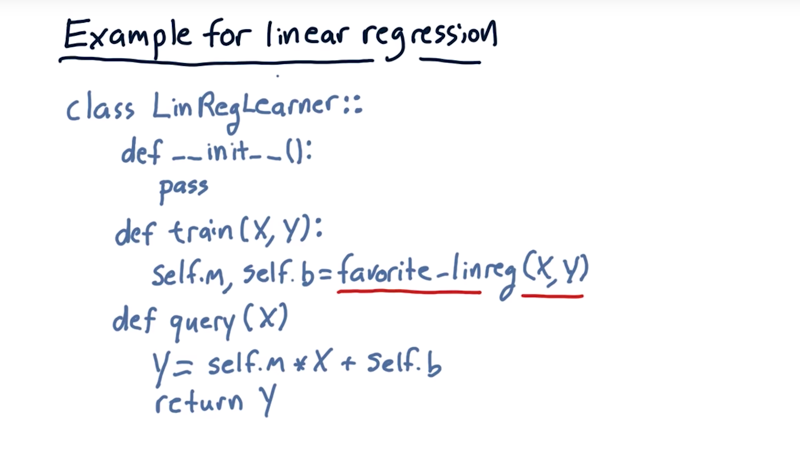pseudo code for implementing the API for a linear regression learner.

Time: 00:02:13

Total Time: 00:18:55

``````2019-01-23 09:13:42
``````